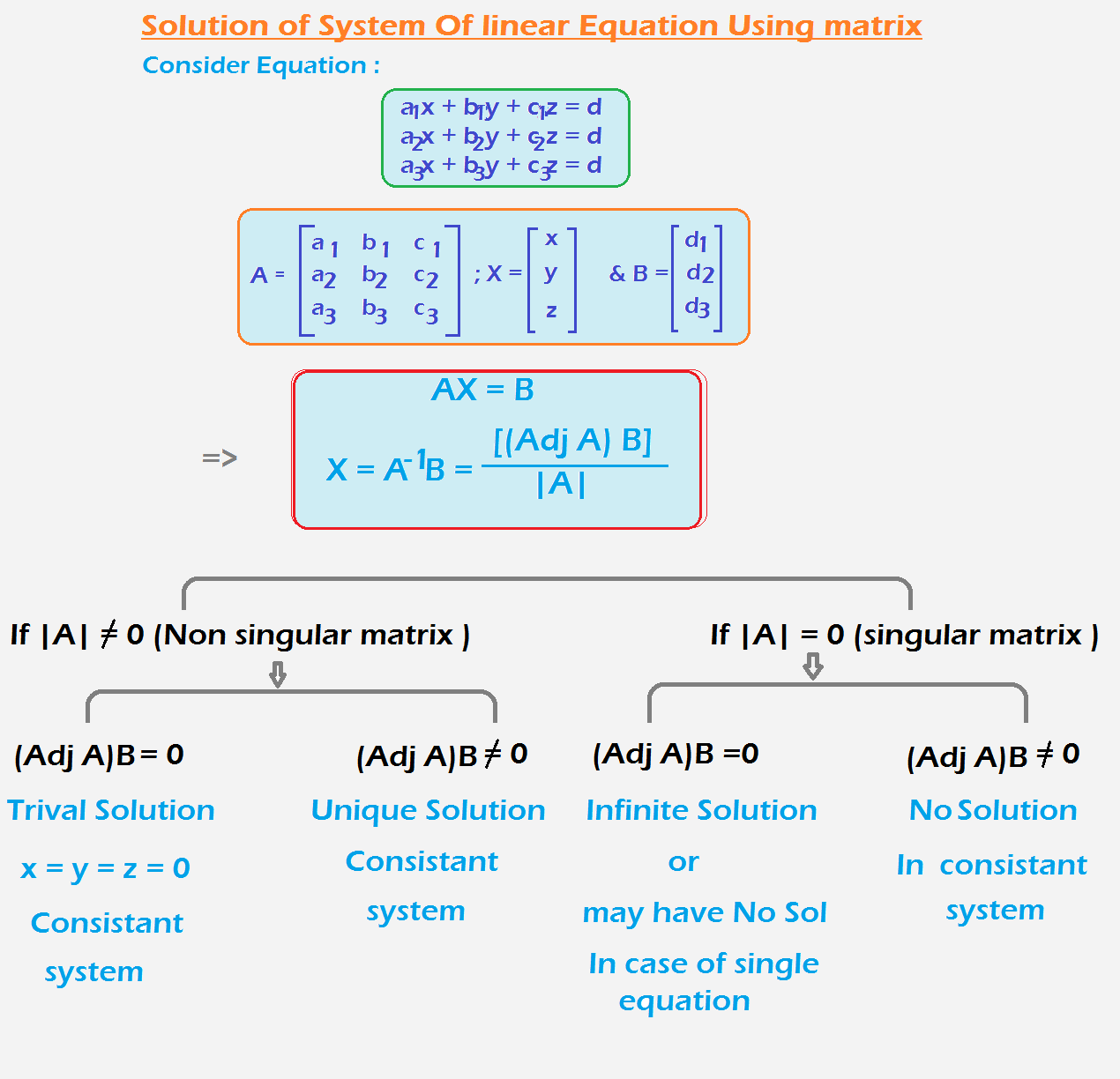Mathematics Revision Of Determinants For CBSE-NCERT
Click for Only Video

### Determinantscolor{green} ✍️ To every square matrix A = [a_(ij)] of order n, we can associate a number (real or complex) called determinant of the square matrix A, where a_(ij) = (i, j)^(th) element of A.

Determinant of a matrix of order one :

A = [a ] be the matrix of order 1, then determinant of A is defined to be equal to color{orange}{|A| = a}

Determinant of a matrix of order 2 × 2 :

=> If A = [(a_(11), a_(12)),(a_(21), a_(22))] be a matrix of order 2 × 2,

then the determinant of A is defined as color{orange}{|A| = |(a_(11), a_(12)),(a_(21), a_(22))|}

Determinant of a matrix of order 3 × 3 :

color{green} ✍️ If A = [(a_(11), a_(12),a_13),(a_(21), a_(22),a_23),(a_31,a_32,a_33)] be a matrix of order 3 × 3,

then the determinant of A is defined as color{orange} |A| = |(a_(11), a_(12),a_13),(a_(21), a_(22),a_23),(a_31,a_32,a_33)|

### Expansion of 3xx3 determinants alog first Row### Properties Of Matrix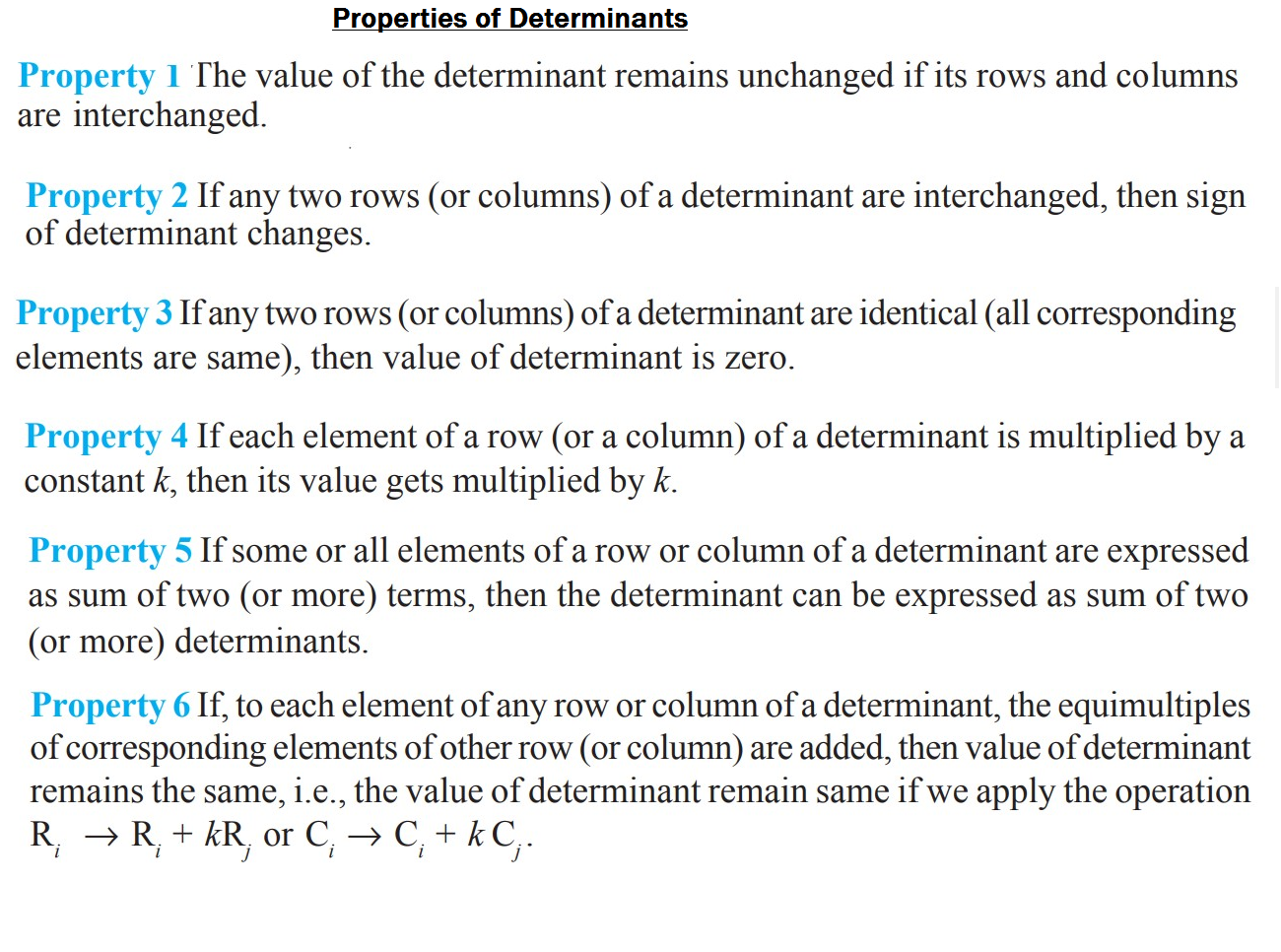### Area Of Triangle

color{green} ✍️ The area of triangle whose vertices are (x_1,y_1) , (x_2,y_2) and (x_3,y_3)

= |1/2[x_1(y_2 -y_3) + x_2(y_3-y_1) + x_3(y_1-y_2)]|

● Now this expression can be written in the form of a determinant as

color{red}{"Area =" 1/2 |(x_1,y_1,1),(x_2,y_2,1),(x_3,y_3,1) |}

### Minors Of Matrix

color{green} ✍️ Minor of an element a_(ij) of a determinant is the determinant obtained by deleting its i^(th) row and j^(th) column in which element a_(ij) lies. Minor of an element a_(ij) is denoted by M_(ij) .### Cofactor of Matrix

color{green} ✍️ Cofactor of an element a_(ij) , denoted by A_(ij) is defined by

=>color{red} {"cofactor of element " a_(ij) "is define as " A_(ij) = (-1)^(i+j)M_(ij)}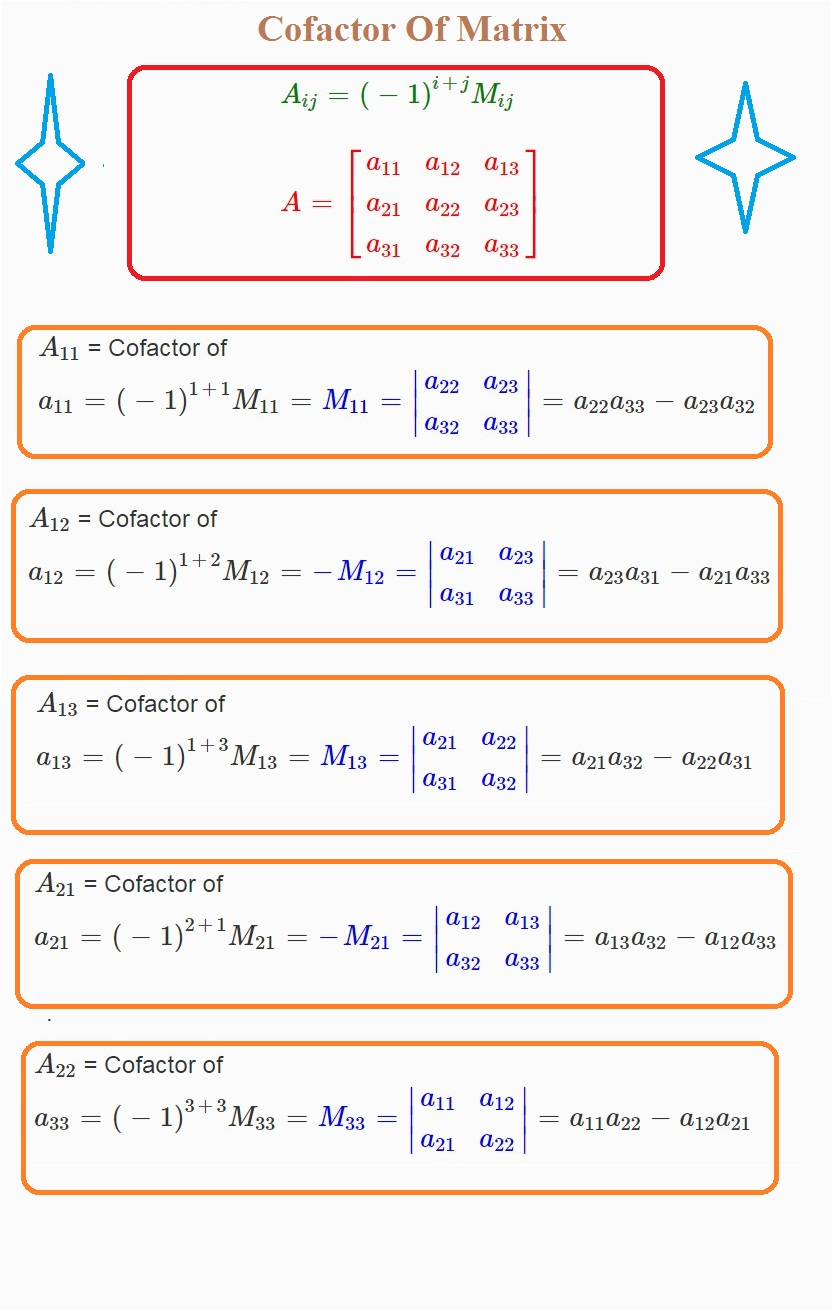### Theorem

Theorem 1 : Sum of Product of any element with their corresponding Cofactor is equal to the value of Determinants.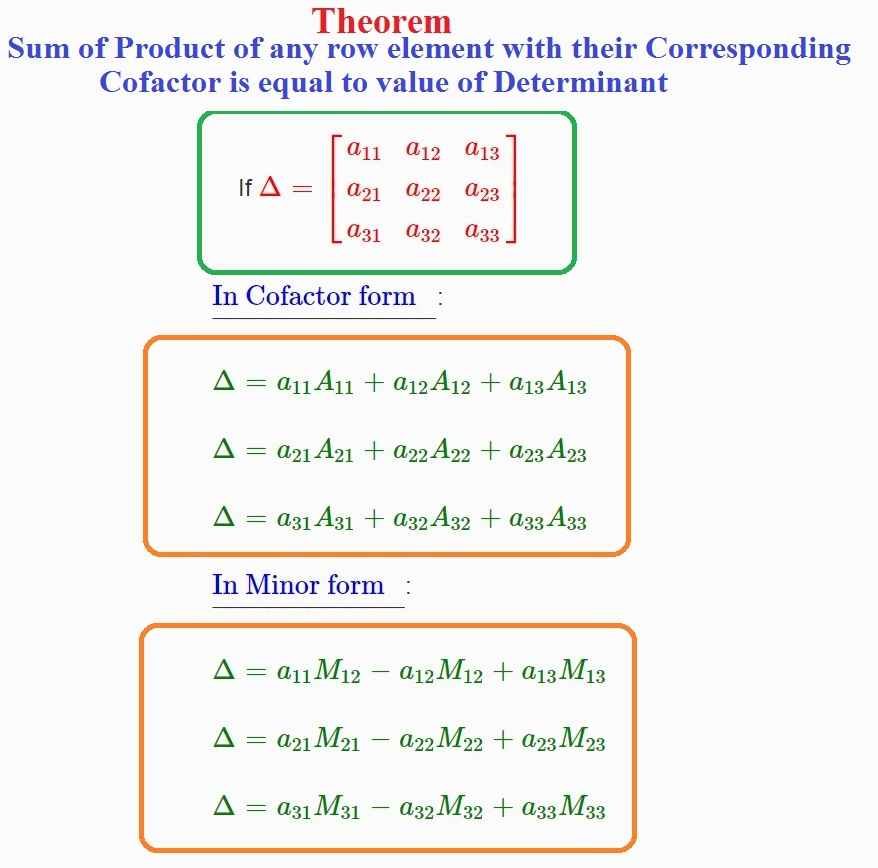Theorem 2 : If elements of a row (or column) are multiplied with cofactors of any other row (or column), then their sum is zero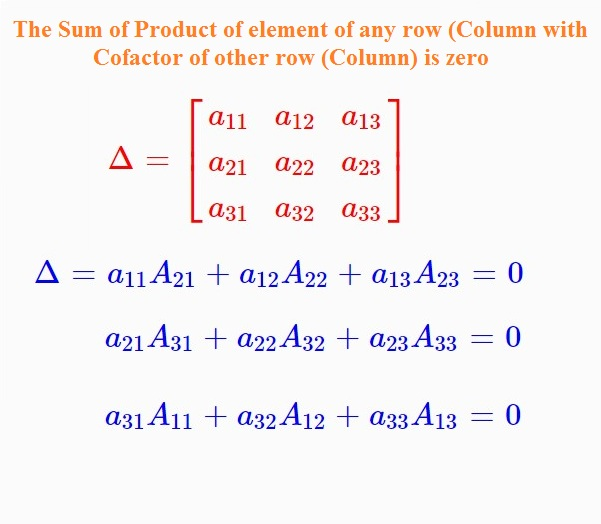### Adjoint of a matrix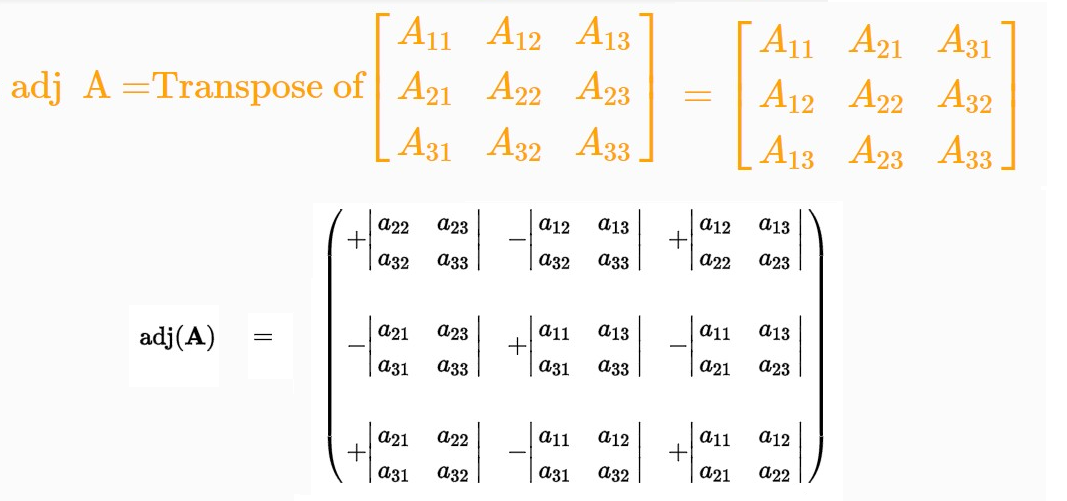\color{green} ✍️ The adjoint of a square matrix A = [a_(ij ) ]_(n × n) is defined as the transpose of the matrix [A_(ij) ]_(n × n),

 => color {red} {A_(ij) " is the cofactor of the element" a_(ij)} . Adjoint of the matrix A is denoted by adj A.

Theorem 1 : color{red}{A(adj A) = (adj A) A = | A | I}

Theorem 2 : color{red}{"If A is a square matrix of order n, then" |adj(A)| = |A|^(n – 1)}.

### Singular And Non-singular Matrix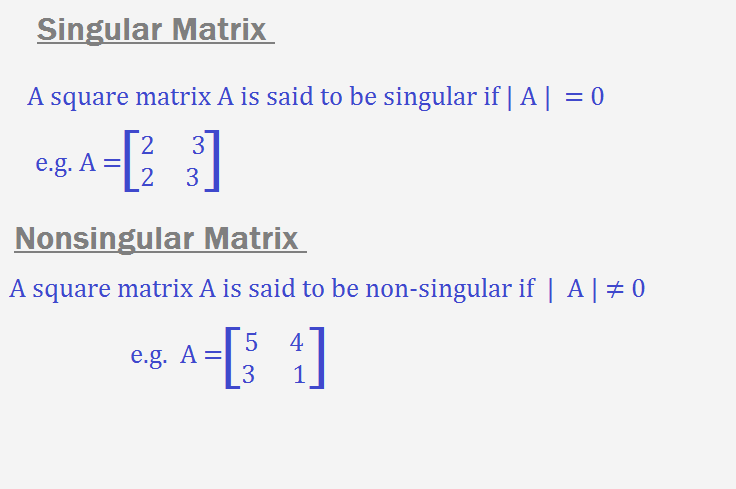"Singular Matrix :"

color{red}{"A square matrix A is said to be singular if" | A | = 0.}

"NonSingular Matrix : "

color{red}{"A square matrix A is said to be non-singular if "| A | ≠ 0}

### Inverse Of Matrix : A^(–1) = 1/(|A|) adj A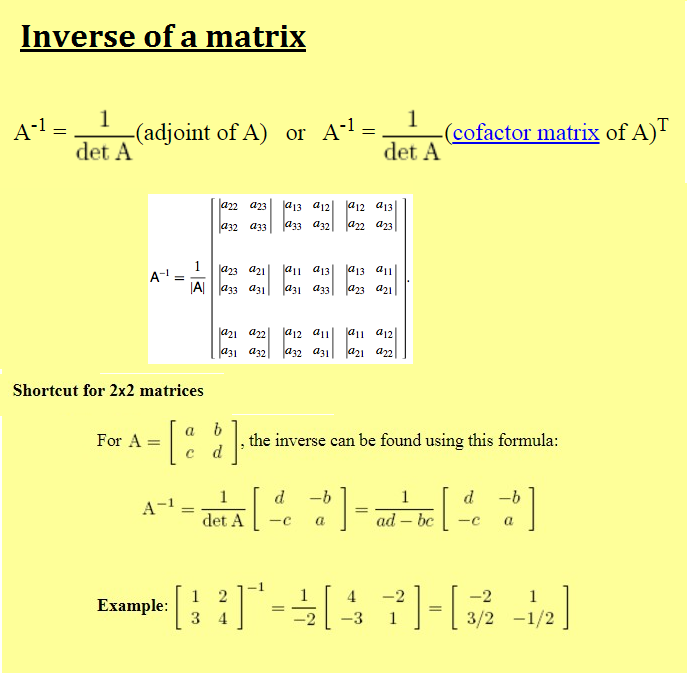color{green}{A^(–1) = 1/(|A|) adj A}

So color{blue}{A^(-1) "exist iff" |A| ne 0}

### Solution of system of linear equations using inverse of a matrix